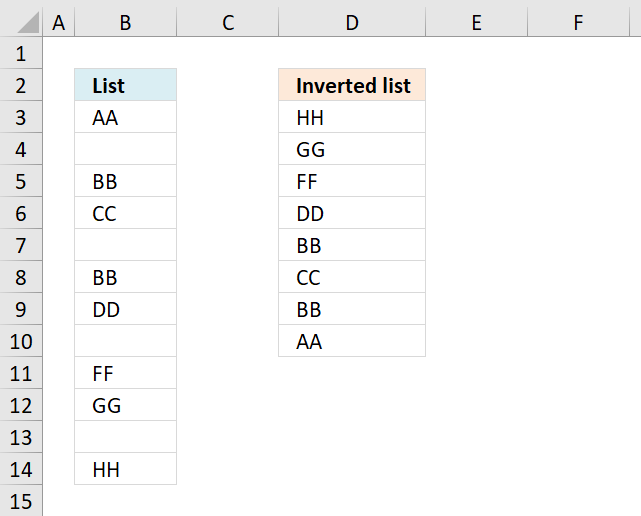Author: Oscar Cronquist Article last updated on November 29, 2018The image above demonstrates a formula in cell D3 that rearranges values, bottom value is now on top etc.

Formula in C2:

=INDEX(\$B\$3:\$B\$14, LARGE(IF(\$B\$3:\$B\$14<>"", MATCH(ROW(\$B\$3:\$B\$14), ROW(\$B\$3:\$B\$14)), ""), ROWS(\$D\$1:D1)))

To enter an array formula, type the formula in a cell then press and hold CTRL + SHIFT simultaneously, now press Enter once. Release all keys.

The formula bar now shows the formula with a beginning and ending curly bracket telling you that you entered the formula successfully. Don't enter the curly brackets yourself.

### Explaining formula in cell D3

#### Step 1 - Find non-empty cells

\$B\$3:\$B\$14<>""

becomes

{"AA"; ""; "BB"; "CC"; ""; "BB"; "DD"; ""; "FF"; "GG"; ""; "HH"}<>""

and returns

{TRUE; FALSE; TRUE; TRUE; FALSE; TRUE; TRUE; FALSE; TRUE; TRUE; FALSE; TRUE}

#### Step 2 - Replace TRUE with corresponding row value

The IF function returns the row number if cell is not blank. FALSE returns "" (nothing).

IF(\$B\$3:\$B\$14<>"", MATCH(ROW(\$B\$3:\$B\$14), ROW(\$B\$3:\$B\$14)), "")

becomes

IF({TRUE; FALSE; TRUE; TRUE; FALSE; TRUE; TRUE; FALSE; TRUE; TRUE; FALSE; TRUE}, MATCH(ROW(\$B\$3:\$B\$14), ROW(\$B\$3:\$B\$14)), "")

becomes

IF({TRUE; FALSE; TRUE; TRUE; FALSE; TRUE; TRUE; FALSE; TRUE; TRUE; FALSE; TRUE}, {1;2;3;4;5;6;7;8;9;10;11;12}, "")

and returns

{1;"";3;4;"";6;7;"";9;10;"";12}

#### Step 3 - Find k-th largest row number

The LARGE function extracts the k-th smallest number from cell range or array. LARGE(array. k) The second argument contains ROWS(\$D\$1:D1), it has an expanding cell reference that grows when cell is copied to cells below.

LARGE(IF(\$B\$3:\$B\$14<>"", MATCH(ROW(\$B\$3:\$B\$14), ROW(\$B\$3:\$B\$14)), ""), ROWS(\$D\$1:D1))

becomes

LARGE({1;"";3;4;"";6;7;"";9;10;"";12}, ROWS(\$D\$1:D1))

becomes

LARGE({1;"";3;4;"";6;7;"";9;10;"";12}, 1)

and returns 12.

#### Step 4 - Return value

The INDEX function returns a value based on a row and column number. In this formula the cell range is only in one column, the row number is only needed.

INDEX(\$B\$3:\$B\$14, LARGE(IF(\$B\$3:\$B\$14<>"", MATCH(ROW(\$B\$3:\$B\$14), ROW(\$B\$3:\$B\$14)), ""), ROWS(\$D\$1:D1)))

becomes

INDEX(\$B\$3:\$B\$14, 12)

and returns "HH" in cell D3.